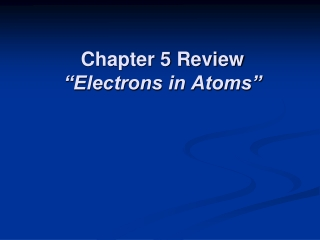DownloadDownload PresentationChapter 5 Review “Electrons in Atoms”

# Chapter 5 Review “Electrons in Atoms”

Télécharger la présentation## Chapter 5 Review “Electrons in Atoms”

- - - - - - - - - - - - - - - - - - - - - - - - - - - E N D - - - - - - - - - - - - - - - - - - - - - - - - - - -
##### Presentation Transcript

1. Chapter 5 Review“Electrons in Atoms”

2. Chapter 5 Review • What is the next atomic orbital in the series: 1s, 2s, 2p, 3s, 3p, ? • In Bohr’s model of the atom, where are the electrons and protons located? • What is the basis for exceptions to the aufbau diagram? • How does the energy of an electron change when the electron moves closer to the nucleus?

3. Chapter 5 Review • If the spin of one electron in an orbital is “clockwise”, what is the spin of the other electron in that orbital? • What is the energy of a photon having a frequency of 4 x 107 Hz? (h = 6.626 x 10-34 J . s) • Which of the following would be most stable: a) 4d55s1, or b) 4d45s2 3x10-26 J

4. Chapter 5 Review • How many unpaired electrons are in a sulfur atom (Z = 16)? • According to the Heisenberg uncertainty principle, if the position of a moving particle is known, what other quantity CANNOT be known? • Which of the following has the highest frequency: a) x-rays, or b) gamma rays Velocity

5. Chapter 5 Review • What is the electron configuration of potassium? • What is the number of electrons in the outermost energy level of an oxygen atom? • How does the speed of visible light compare with the speed of gamma rays, when both speeds are measured in a vacuum?

6. Chapter 5 Review • The principal quantum number indicates what property of an electron? • Which scientist developed the quantum mechanical model of the atom? • In the Quantum Mechanical model of the atom, an electron in an orbit has a fixed ___.

7. Chapter 5 Review • What is the maximum number of electrons in the second principal energy level? • If three electrons are available to fill three empty 2p atomic orbitals, how will the electrons be distributed? • What is the wavelength of a wave that has a frequency of 60 Hz? 5x106 m

8. Chapter 5 Review • When an electron moves from a lower to a higher energy level, the electron _____. • According to the aufbau principle, electrons enter orbitals of ___ first. • What is the maximum number of “f” orbitals in any single energy level of an atom?

9. Chapter 5 Review • What is the frequency of a photon having an energy 5 x 10-24 J? (h = 6.626 x 10-34 J . s) 8x109/s • What types of atomic orbitals (s, p, d, or f) are in the third principal energy level?

10. Chapter 5 Review • How many energy sublevels are in the second principal energy level? • Emission of light from an atom occurs when an electron _____. • What are quanta of light called?

11. Chapter 5 Review • Stable electron configurations are likely to contain ____. • What is the maximum number of “d” orbitals in a principal energy level? • How are frequency and wavelength of light related? • What is the maximum number of orbitals in the p sublevel?

12. Chapter 5 Review • Which electron configuration would be more stable: a) 4f7, or b) 4f14 • Which variable is directly proportional to frequency: a) energy, or b) wavelength? • Which color of visible light has the shortest wavelength?

13. Chapter 5 Review • How many electrons are in the highest occupied energy level of a neutral atom of each of the following: a) chlorine atom; b) copper atom; c) strontium atom? End of Chapter 5 Review